Surds & Indices - Introduction and Examples (with Solutions)

# Surds & Indices - Introduction and Examples (with Solutions) Notes | Study Quantitative Aptitude for Competitive Examinations - Banking Exams

## Document Description: Surds & Indices - Introduction and Examples (with Solutions) for Banking Exams 2022 is part of Quantitative Aptitude for Competitive Examinations preparation. The notes and questions for Surds & Indices - Introduction and Examples (with Solutions) have been prepared according to the Banking Exams exam syllabus. Information about Surds & Indices - Introduction and Examples (with Solutions) covers topics like and Surds & Indices - Introduction and Examples (with Solutions) Example, for Banking Exams 2022 Exam. Find important definitions, questions, notes, meanings, examples, exercises and tests below for Surds & Indices - Introduction and Examples (with Solutions).

Introduction of Surds & Indices - Introduction and Examples (with Solutions) in English is available as part of our Quantitative Aptitude for Competitive Examinations for Banking Exams & Surds & Indices - Introduction and Examples (with Solutions) in Hindi for Quantitative Aptitude for Competitive Examinations course. Download more important topics related with notes, lectures and mock test series for Banking Exams Exam by signing up for free. Banking Exams: Surds & Indices - Introduction and Examples (with Solutions) Notes | Study Quantitative Aptitude for Competitive Examinations - Banking Exams
 1 Crore+ students have signed up on EduRev. Have you?

What are Surds?

The roots of those quantities which cannot be exactly obtained are called Surds.

Examples: √3, 2√5, 7√2

What are Indices?

The expression 25 is defined as follows:

25 = 2 × 2 × 2 × 2 × 2

We call "2" the base and "5" the index.

Shortcut to Remember Surds & Indices: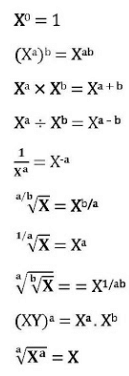1. If √(X/0.0081) = ∛0.009 , the value of x is:
a) 0.729
b) 0.0729
c) 0.000729
d) 0.00729
e) None of these

### Solution:

√(X/0.0081) = ∛0.009
Or, √x/0.09 = ∛(9/1000)
Or, √x = 0.09 × 3/10
Or, √x = 0.027
Or, x = (0.027)2 = 0.000729

So, answer is option c.

2. The value of √(9+ √(604+ √(424+ √(273+ √256) ) ) ) = ?
a) 8
b) 6
c) 5
d) 7
e) None of these

### Solution:

√(11+ √(604+ √(424+ √(273+ √256) ) ) )
= √(11+ √(604+ √(424+ √(273+ 16)) ) )
= √(11+ √(604+ √(424+ √289) ) )
= √(11+ √(604+ √(424+ 17)) )
= √(11+ √(604+ √441) )
= √(11+ √(604+ 21))
= √(11+ √625)
= √(11+ 25)
= √(11+ 25)
= √36
= 6

So, answer is option b.

3. Simplification: 252.7 × 54.2 ÷ 55.4 = ?

a) 54
b) 53.2
c) 54.1
d) 54.2
e) None of these

### Solution:

252.7 × 54.2 ÷ 55.4
= 55.4 × 54.2 ÷ 55.4
= 55.4 × 54.2 ÷ 55.4
= 55.4 × 5-1.2
= 5(5.4 -1.2)
= 54.2
So, answer is option d.

4. (625)0.16 × (625)0.09 = ?
a) 125
b) 25
c) 6.25
d) 12.5
e) 5

Solution:

(625)0.16 × (625)0.09

= (625)0.16 + 0.09

= (625)0.25

= (625)1/4

= 54 × ¼

= 5

So, answer is option e.

1. Laws of Indices:

1. amanam +
2.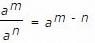3. (am)namn
4. (ab)nanbn
5.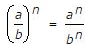6. a0 = 1

2. Surds:

Let a be rational number and n be a positive integer such that a(1/n)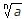Then, a is called a surd of order n.

3. Laws of Surds: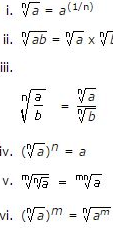The document Surds & Indices - Introduction and Examples (with Solutions) Notes | Study Quantitative Aptitude for Competitive Examinations - Banking Exams is a part of the Banking Exams Course Quantitative Aptitude for Competitive Examinations.
All you need of Banking Exams at this link: Banking Exams

## Quantitative Aptitude for Competitive Examinations

33 videos|34 docs|140 tests
 Use Code STAYHOME200 and get INR 200 additional OFF

## Quantitative Aptitude for Competitive Examinations

33 videos|34 docs|140 tests

Track your progress, build streaks, highlight & save important lessons and more!

,

,

,

,

,

,

,

,

,

,

,

,

,

,

,

,

,

,

,

,

,

;